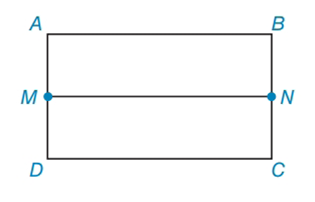Chapter 4.3, Problem 7EElementary Geometry For College St...

7th Edition
Alexander + 2 others
ISBN: 9781337614085

Solutions

Chapter
SectionElementary Geometry For College St...

7th Edition
Alexander + 2 others
ISBN: 9781337614085
Textbook Problem

A line segment joins the midpoints of two opposite sides of a rectangle as shown. What can you conclude regarding M N ¯ , A B ¯ , D C ¯  and  M N , A B , D C ?To determine

To find:

The conclusion regarding MN¯,AB¯,DC¯ and MN,AB,DC.

Explanation

Calculation:

Given: A line segment joins the midpoints of two opposite sides of a rectangle as shown.

This problem shows the rectangle ABCD with points labelled and M, N as the midpoints of two opposite sides. A line segment MN such that M divides AD in the middle and N divides BC in the middle...

Still sussing out bartleby?

Check out a sample textbook solution.

See a sample solution

The Solution to Your Study Problems

Bartleby provides explanations to thousands of textbook problems written by our experts, many with advanced degrees!

Get Started

Factoring Factor the expression completely. 27. x4 2x2 + 1

Precalculus: Mathematics for Calculus (Standalone Book)

let f(x) = x 1, g(x) = x+1, and h(x) = 2x3 1. Find the rule for each function. 11. fg

Applied Calculus for the Managerial, Life, and Social Sciences: A Brief Approach

Solve each equation for if 0360. 2csc=2

Trigonometry (MindTap Course List)

True or False: By the Integral Test, converges.

Study Guide for Stewart's Multivariable Calculus, 8th